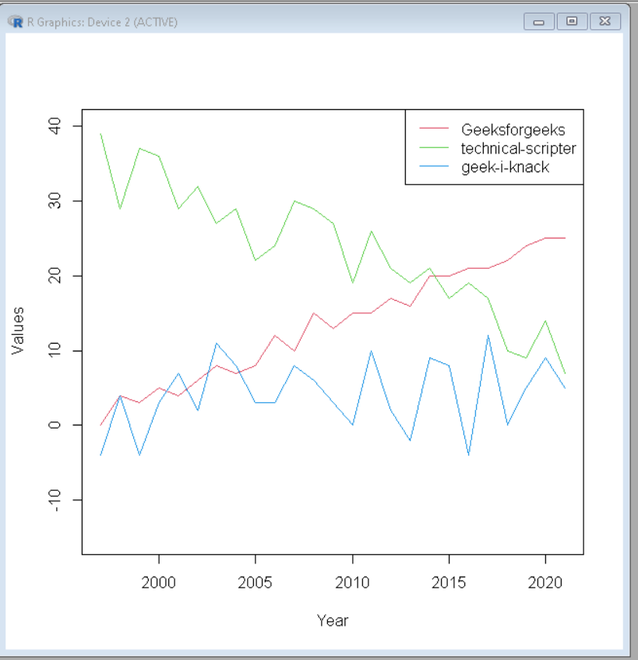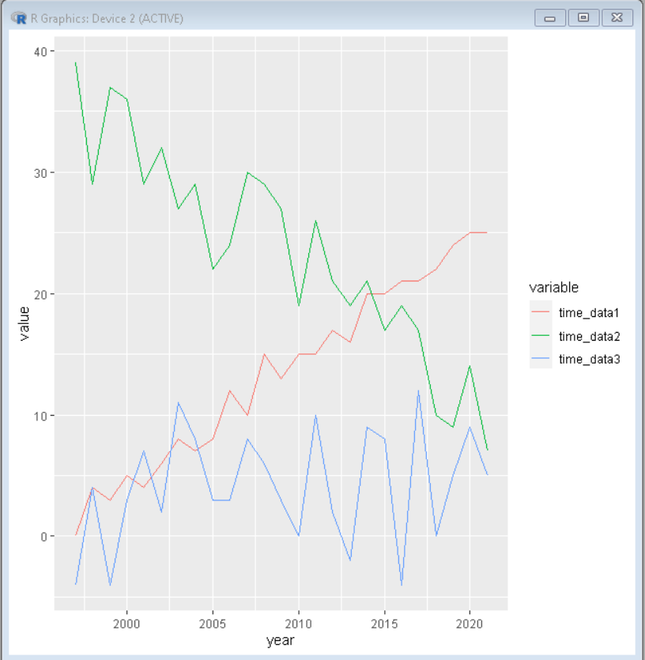Related Articles

# Draw Multiple Time Series in Same Plot in R

• Difficulty Level : Expert
• Last Updated : 17 Jun, 2021

Time Series in R programming language is used to see how an object behaves over a period of time. In R, it can be easily done by the ts() function with some parameters. Time series takes the data vector and each data is connected with timestamp value as given by the user.

### Method 1: Using Basic R methods

First, we create a data vector that has data for all the time series that have to be drawn. Then we plot the time series using the first dataset and plot() function. Then add other time series using line() function to the existing plot. Then we may add a legend on top to show which line in the plot represents which time series.

Example:

## R

 `# Create sample data``set.seed``(1023)                           `` ` `sample_data <- ``round``(``data.frame``(year = 1997:2021,``                     ``time_data1 = 1:25 + ``rnorm``(25),``                     ``time_data2  = 30:6 + ``runif``(25, 0, 10),``                     ``time_data3  = ``rnorm``(25, 5, 5)))`` ` `# Plot a graph with time_data1``plot``(sample_data\$year,                           ``     ``sample_data\$time_data1,``     ``type = ``"l"``,``     ``col = 2,``     ``ylim = ``c``(- 15, 40),``     ``xlab = ``"Year"``,``     ``ylab = ``"Values"``)`` ` `# Add line graphs of other two dataset``lines``(sample_data\$year,                             ``      ``sample_data\$time_data2,``      ``type = ``"l"``,``      ``col = 3)`` ` `lines``(sample_data\$year,                             ``      ``sample_data\$time_data3,``      ``type = ``"l"``,``      ``col = 4)`` ` `# Add legend in top right corner``legend``(``"topright"``,                           ``       ``c``(``"Geeksforgeeks"``, ``"technical-scripter"``, ``"geek-i-knack"``),``       ``lty = 1,``       ``col = 2:4)`

Output:Multiple time series in one plot

### Method 2: Using ggplot2

In ggplot2 we can directly use geom_line() to draw the plot.

We first create a sample data vector. The ggplot2 will be used to draw the plot and reshape will melt the data from the wide form to long-form. Convert data from the wide form to long-form.

Syntax:

melt(sample_data, id.vars = variable)

Draw the plot with the help of ggplot2 and geom_line(),

Example:

## R

 `# Create sample data``set.seed``(1023)                           `` ` `sample_data <- ``round``(``data.frame``(year = 1997:2021,``                     ``time_data1 = 1:25 + ``rnorm``(25),``                     ``time_data2  = 30:6 + ``runif``(25, 0, 10),``                     ``time_data3  = ``rnorm``(25, 5, 5)))`` ` `# Load packages reshape2 and ggplot2``library``(``"reshape2"``)  ``library``(``"ggplot2"``)   `` ` `# Convert sample_data from wide form to long form``data_final <- ``melt``(sample_data, id.vars = ``"year"``)`` ` `# Plot the dinal data``ggplot``(data_final,                            ``       ``aes``(x = year,``           ``y = value,``           ``col = variable)) + ``geom_line``()`

Output:Multiple time series using ggplot2

My Personal Notes arrow_drop_up